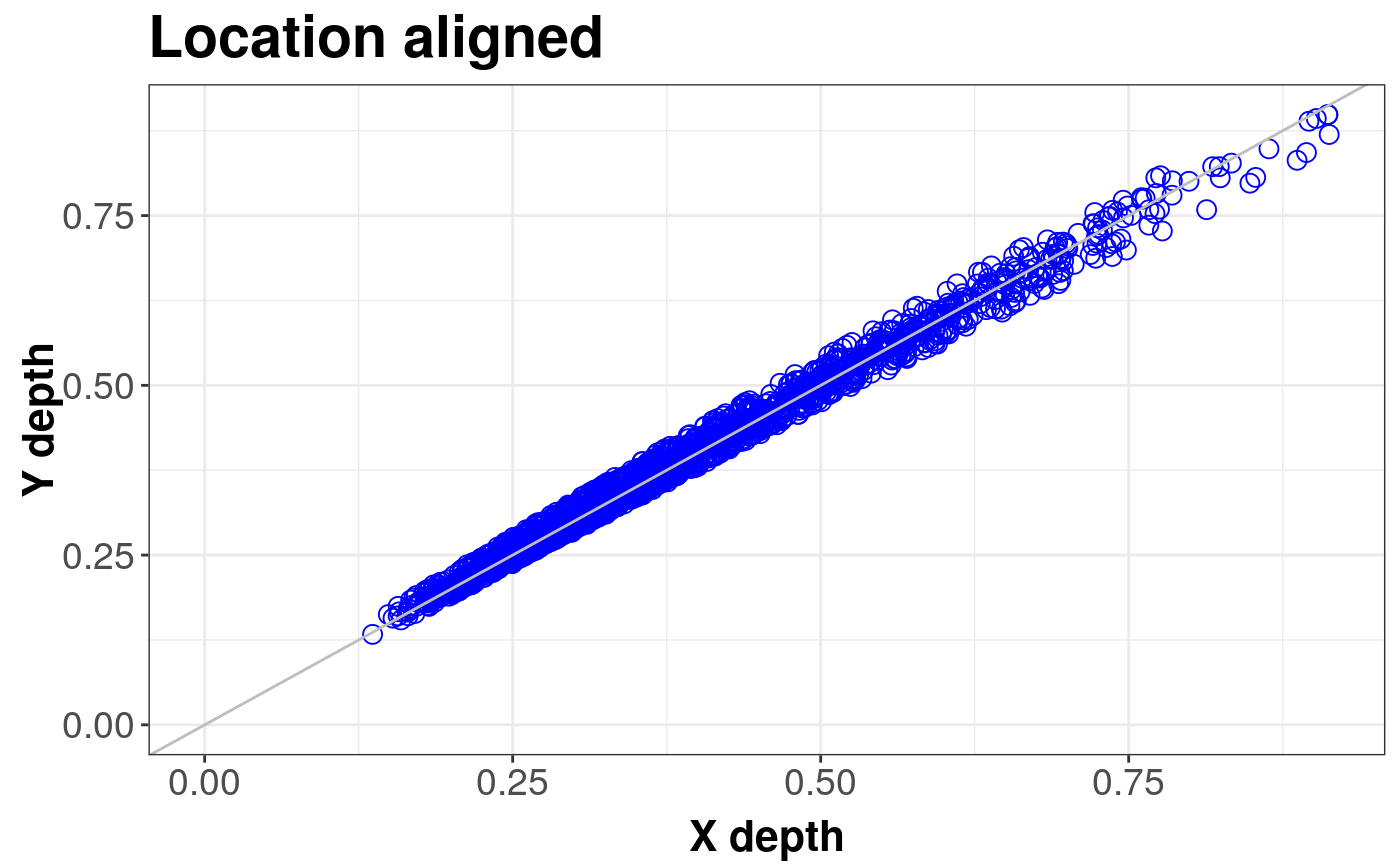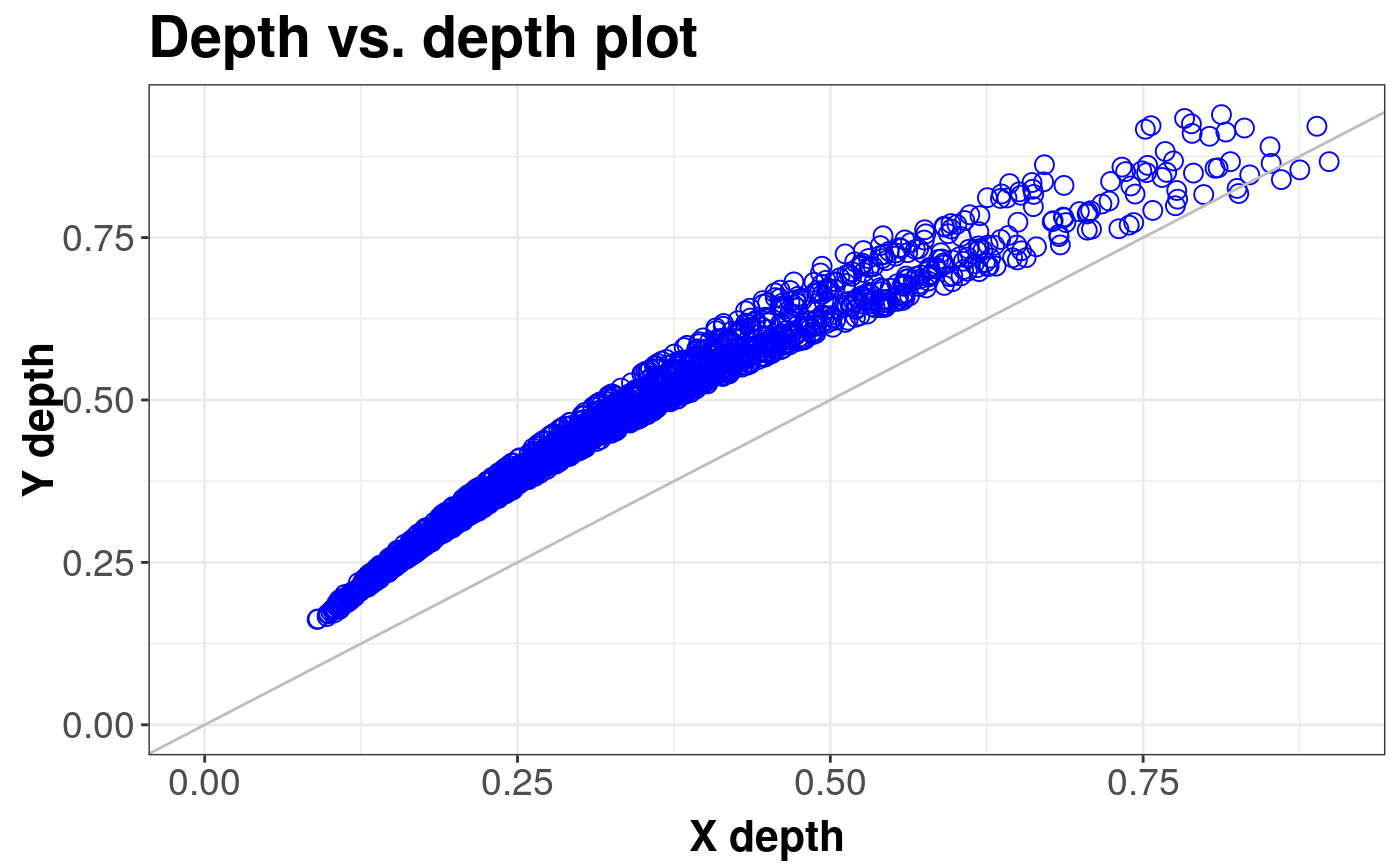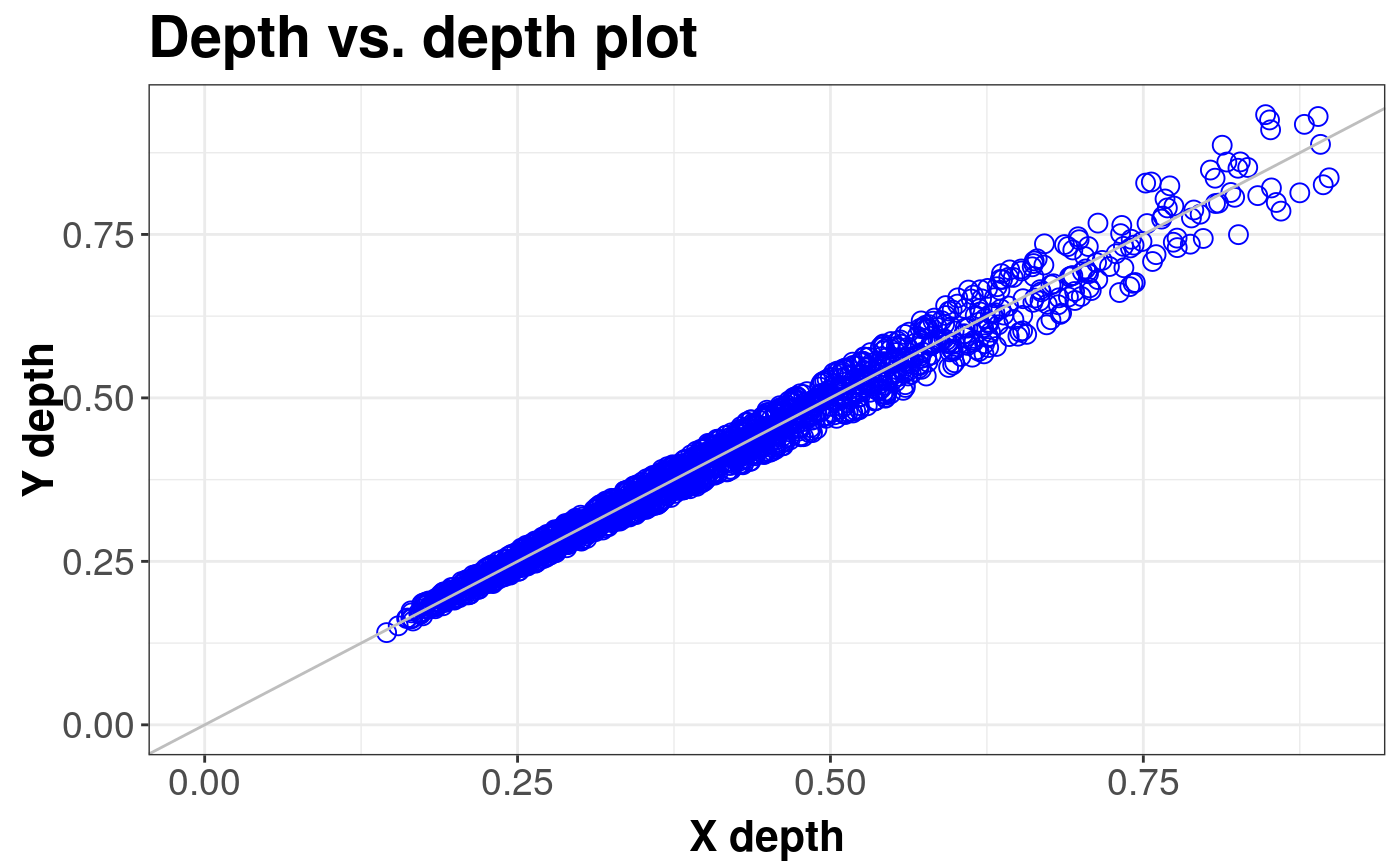Produces a DD plot which allows to compare two multivariate datasets or to compare a subject dataset with theoretical distribution.

ddPlot(x, y, scale = FALSE, location = FALSE, name = "X",
name_y = "Y", title = "Depth vs. depth plot",
depth_params = list())

## Arguments

x The first or only data sample for ddPlot. The second data sample. x and y must be of the same space. logical. determines whether the dispersion is to be aligned. determines whether the location is to be aligned to 0 vector with depth median. name for data set x. It will be passed to drawing function. as above for y title of the plot. list of parameters for function depth (method, threads, ndir, la, lb, pdim, mean, cov, exact).

## Details

For two probability distributions $$F$$ and $$G$$, both in $${{{R}} ^ {d}}$$, we can define depth vs. depth plot being very useful generalization of the one dimensional quantile-quantile plot: $$DD(F, G) = \left\{\left( D({z}, F), D({z}, G) \right), {z} \in {{{R}} ^ {d}} \right\}$$ Its sample counterpart calculated for two samples $${{{X}} ^ {n}} = \{{{X}_{1}}, ..., {{X}_{n}}\}$$ from $$F$$, and $${{Y} ^ {m}} = \{{{Y}_{1}}, ..., {{Y}_{m}}\}$$ from $$G$$ is defined as $$DD({{F}_{n}}, {{G}_{m}}) = \left\{\left( D({z}, {{F}_{n}}), D({z}, {{G}_{m}}) \right), {z} \in \{{{{X}} ^ {n}} \cup {{{Y}} ^ {m}}\} \right\}$$

Liu, R.Y., Parelius, J.M. and Singh, K. (1999), Multivariate analysis by data depth: Descriptive statistics, graphics and inference (with discussion), Ann. Statist., 27, 822--831.

Liu, R.Y., Singh K. (1993), A Quality Index Based on Data Depth and Multivariate Rank Test, Journal of the American Statistical Association vol. 88.

## Examples

library(sn)
library(mvtnorm)

# EXAMPLE 1: Location difference
standard <- mvrnorm(1000, c(0, 0), diag(2))
shift <- mvrnorm(1000, c(0.5, 0), diag(2))
ddPlot(x = standard, y = shift, title = "Difference in position")#> DDPlot#>
#> Depth Metohod:
#> 	 ProjectionddPlot(x = standard, y = shift, location = TRUE, title = "Location aligned")#> DDPlot#>
#> Depth Metohod:
#> 	 Projection
# EXAMPLE 2: Scale difference
standard <- mvrnorm(1000, c(0, 0), diag(2))
scale <- mvrnorm(1000, c(0, 0), 4 * diag(2))
ddPlot(x = standard, y = scale)#> DDPlot#>
#> Depth Metohod:
#> 	 ProjectionddPlot(x = standard, y = scale, scale = TRUE)#> DDPlot#>
#> Depth Metohod:
#> 	 Projection